Maths-
General
Easy

Question

# If P (q) and Q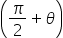are two points on the ellipse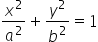, then locus of the mid – point of PQ is

##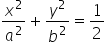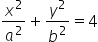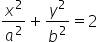none of theseHint:

## The correct answer is:### Coordinate of Point P(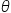) = (a cos, b sin)Coordinate of Point Q (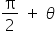) = ( a cos() ,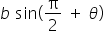)Coordinate of Point Q () = ( -a sin(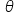) ,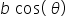)Let R be the midpoint of P and Q and coordinates of R = (h, k)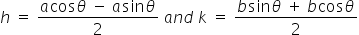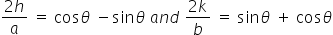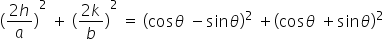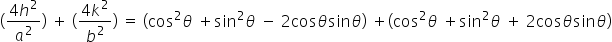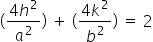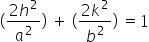comparing with equation of ellipse i.e.#### With Turito Foundation.#### Get an Expert Advice From Turito.